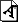# The Continuum Hypothesis: A Mystery of Mathematics?

The concept of infinity has been a subject of speculation throughout history. In the nineteenth century, Georg Cantor produced an elegant theory in which the notion of infinity could be treated mathematically: set theory. The concepts investigated in set theory are the building blocks on which the whole of mathematics can be built. In set theory the size of an infinite set can be measured by its cardinality.

Many problems regarding cardinal numbers that have a simple formulation are unsolved even after years of effort by outstanding mathematicians. The continuum problem asks for a solution of the continuum hypothesis (CH), and is the first in Hilbert's celebrated list of 23 problems. This problem has an ambivalent nature: it is comprehensible to a vast community of scientists, but the effort devoted to solving it shows that even in mathematics there are deceptively simple questions which may not have an answer. More precisely, the existence of 'solutions' depends on the philosophical attitude of the person seeking them: does every precisely stated mathematical question have a solution? Most Platonists believe so, and a large number of them believe that the latest research in set theory is leading to a satisfactory solution of the continuum problem in particular. A skeptical mathematician, on the other hand, is apt to believe that this problem will never be settled.

While there are many ways to formulate CH, we choose the following:

For every subset X of the real numbers, either there is an injective map from X into the natural numbers or there is an injective map from the real numbers into X.

Gödel and Cohen showed that the accepted axioms of set theory cannot solve CH. Gödel in 1939 was able to produce a model of set theory in which CH is true. In work for which he was awarded the Fields Medal, Cohen in 1963 introduced the forcing method and used it to produce a model of set theory in which CH is false.

While many set theorists (including Cohen himself) are convinced that these results proved that the continuum problem could never be settled, many others (including Gödel) believed that they merely showed that new 'natural axioms' of set theory powerful enough to settle the continuum problem should be sought. In the spirit of the latter approach, a major achievement during the eighties was the proof by Martin, Steel and Woodin that there is a 'true' axiom (projective determinacy), which implies that any counter-example to CH must be very complicated  essentially 'indefinable' in a technical sense.

Recent research aims at isolating 'maximality properties': principles which say that the greatest possible variety of conceivable mathematical objects exists. A 'natural' class of axioms that approximate this criterion, the so-called 'forcing axioms', have been found. These imply the axiom of projective determinacy and the failure of CH, as well as many new and surprising results in general topology and infinite combinatorics. Hugh Woodin has provided a philosophical motivation for the failure of CH: he has solid mathematical arguments to conjecture that any 'maximality principle' able to settle all the problems of mathematics of the same 'complexity' as CH will imply the failure of CH.

Forcing axioms solve another problem in cardinal arithmetic of a 'higher complexity' than CH: the singular cardinal hypothesis (SCH) asserts that the cardinality of the power-set of strong limit cardinals is the least possible. SCH is true in Gödel's model, and models of set theory where SCH is false can be produced only by combining forcing techniques with the use of large cardinals. In my thesis I was able to generalize a result of Solovay in order to prove that a 'popular' forcing axiom, the proper forcing axiom (PFA), implies SCH.

There are grounds to conjecture that SCH is just an example of the class of problems of 'higher complexity' than CH that can be settled by forcing axioms. The correct definition of this class of problems as well as the search for the solutions given by forcing axioms is a topic of active research in set theory.

Matteo Viale has been awarded the 2006 Sacks Prize. The Sacks Prize is awarded by the Association for Symbolic Logic for the most outstanding doctoral dissertation in mathematical logic. It was established to honor Professor Gerald Sacks of MIT and Harvard for his unique contribution to mathematical logic, particularly as adviser to a large number of excellent Ph.D. students.

E-mail: matteologic.univie.ac.at